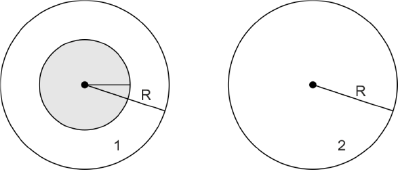#### A uniform magnetic field is restricted within a region of radius r. The magnetic field changes with time at a rate. Loop 1 of radius R > rencloses the region r and loop 2 of radius R is outside the region of magnetic field as shown in the figure below. Then the e.m.f. generated isOption 1) zero in loop 1 and zero in loop 2 Option 2)in loop 1 andin loop 2 Option 3)in loop 1 and zero in loop 2 Option 4)in loop 1 and zero in loop 2As discussed in

Rate of change of magnetic Flux -- whereinchange in flux

In loop 1,In loop 2,Option 1)

zero in loop 1 and zero in loop 2

This option is incorrect.

Option 2)in loop 1 andin loop 2

This option is incorrect.

Option 3)in loop 1 and zero in loop 2

This option is incorrect.

Option 4)in loop 1 and zero in loop 2

This option is correct.

#### Plabita